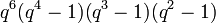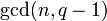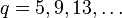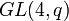# Element structure of special linear group of degree four over a finite field

## Contents

This article gives specific information, namely, element structure, about a family of groups, namely: special linear group of degree four.
View element structure of group families | View other specific information about special linear group of degree four

This article describes the element structure of the special linear group of degree four over a finite field.

We take$q$ as the number of elements in the field and$p$ as the underlying prime number, so$q$ is a power of$p$.

## Summary

Item Value
number of conjugacy classes Case$q$ is even (e.g.,$q = 2,4,8,16,\dots$):$q^3 + q^2 + q = q(q^2 + q + 1)$
Case$q \equiv -1 \pmod 4$ (e.g.,$q = 3,7,11,19,\dots$):$q^3 + q^2 + 4q + 3$
Case$q \equiv 1 \pmod 4$ (e.g.,$q = 5,9,13,17,25,\dots$):$q^3 + q^2 + 4q + 15$
order$q^6(q^4 - 1)(q^3 - 1)(q^2 - 1)$
Factored form:$q^6(q - 1)^3(q + 1)^2(q^2 + 1)(q^2 + q + 1)$
exponent  ?

## Conjugacy class structure

### Number of conjugacy classes

As we know in general, number of conjugacy classes in special linear group of fixed degree over a finite field is PORC function of field size, the degree of this PORC function is one less than the degree of matrices, and we make cases based on the congruence classes modulo the degree of matrices. Thus, we expect that the number of conjugacy classes is a PORC function of the field size of degree 4 - 1 = 3, and we need to make cases based on the congruence class of the field size modulo 4. Moreover, the general theory also tells us that the polynomial function of$q$ depends only on the value of$\operatorname{gcd}(n,q-1)$, which in turn can be determined by the congruence class of$q$ mod$n$ (with$n = 4$ here).

Value of$\operatorname{gcd}(4,q-1)$ Corresponding congruence classes of$q$ mod 4 Number of conjugacy classes (polynomial of degree 4 - 1 = 3 in$q$) Additional comments
1 0 or 2 mod 4 (e.g.,$q = 2,4,8,\dots$)$q^3 + q^2 + q = q(q^2 + q + 1)$ In this case, we have an isomorphism between linear groups when degree power map is bijective, so$SL(4,q) \cong PGL(4,q) \cong PSL(4,q)$
2 3 mod 4 (e.g.,$q = 3,7,11,\dots$)$q^3 + q^2 + 4q + 3$
4 1 mod 4 (e.g.,$q = 5,9,13,\dots$)$q^3 + q^2 + 4q + 15$

### General strategy and summary

Before making the entire table, we recall the general strategy: first, imitate the procedure of element structure of general linear group of degree four over a finite field to determine that$GL(4,q)$-conjugacy classes in$SL(4,q)$. Then, use the splitting criterion for conjugacy classes in the special linear group to determine which of these conjugacy classes split, and how much.

In this case, the fact that conjugacy class of elements with semisimple generalized Jordan block does not split in special linear group over a finite field rules out the possibility of splitting for most conjugacy classes. The following conjugacy classes deserve more investigation. In each case, the details regarding splitting depend on the field size mod 4, or more specifically, on the number$\operatorname{gcd}(4,q-1)$:

• Conjugacy classes with a single size four Jordan block
• Conjugacy classes with two size two Jordan blocks with the same eigenvalue
• Conjugacy classes with two size two Jordan blocks with distinct eigenvalues
• Conjugacy classes with a generalized Jordan block of a$2 \times 2$ type